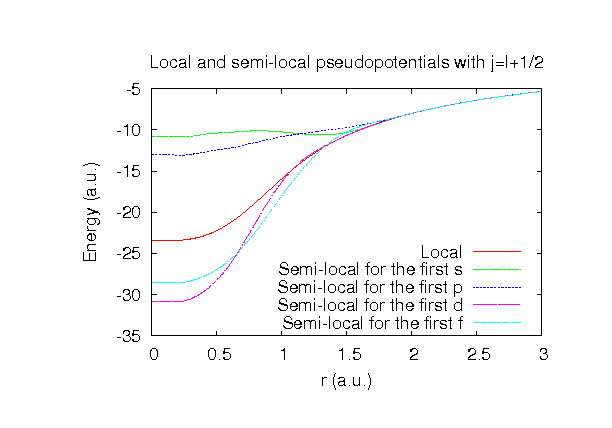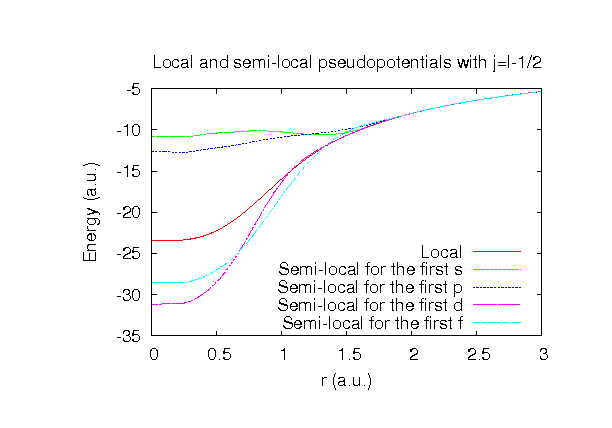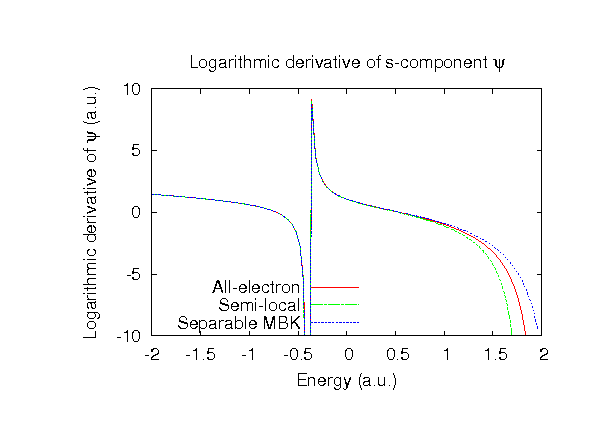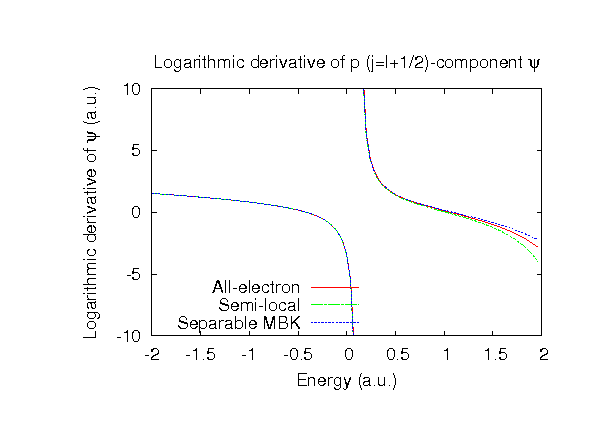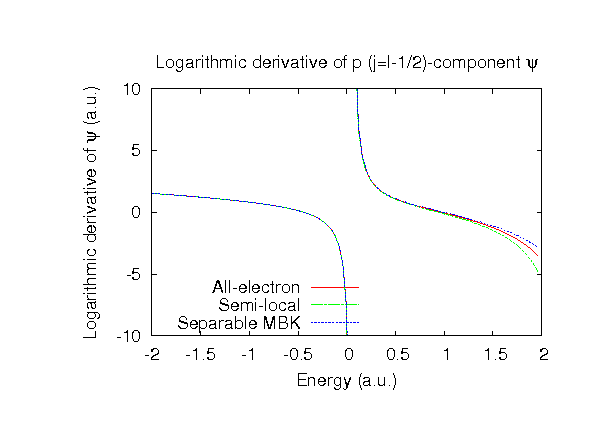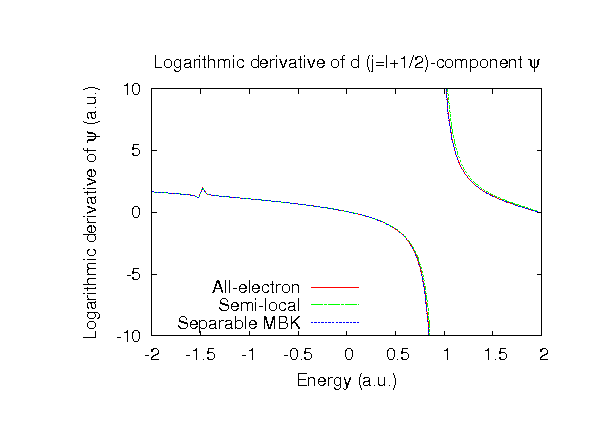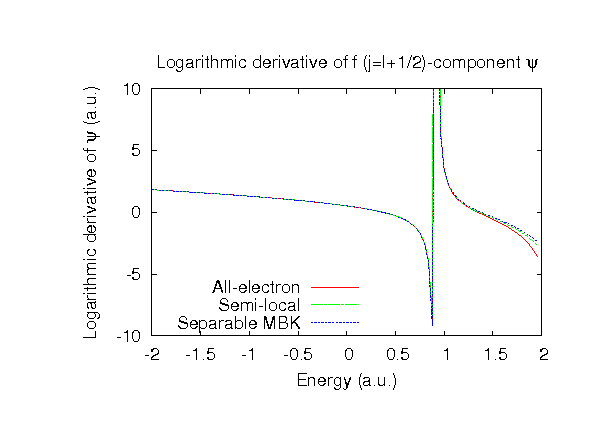### Fully relativistic pseudopotentials

Fully relativistic pseudopotentials generated by the MBK (PRB 47, 6728 (1993)) scheme within LDA (CA13) and GGA (PBE13) which contain a partial core correction and fully relativistic effects including spin-orbit coupling.

### Pseudo-atomic orbitals

The number below the symbol means a cutoff radius (a.u.) of the confinement potential. These file includes fifteen radial parts for each angular momentum quantum number l (=0,1,2,3). The basis functions were generated by variationally optimizing the corresponding primitive basis functions in the single atom, the dimer molecule, and the BCC structure. The input files used for the orbital optimization can be found at Te_opt.dat , Te2_opt.dat , and Tebcc_opt.dat . Since Te_CA13.vps and Te_PBE13.vps include the 4d, 5s, and 5p states (16 electrons) as the valence states, the minimal basis set is Te*.*-s1p1d1. Our recommendation for the choice of cutoff radius of basis functions is that Te7.0.pao is enough for bulks, but Te9.0.pao or Te11.0.pao is preferable for molecular systems.

### Benchmark calculations by the PBE13 pseudopotential with the various basis functions

(1) Calculation of the total energy as a function of lattice constant in the BCC structure, where the total energy is plotted relative to the minimum energy for each case. a0 and B0 are the equilibrium lattice constant and bulk modulus obtained by fitting to the Murnaghan equation of state. The difference between Te7.0-s3p3d2f1 and Te7.0-s4p3d3f2 in the total energy at the minimum point is 0.0608 eV/atom. An input file used for the OpenMX calculations can be found at Tebcc-EvsV.dat . For comparison the result by the Wien2k code is also shown, where the calculation was performed by default setting in the Ver. 9.1 of Wien2k except for the use of RMT x KMAX of 10.(2) Calculations of the band dispersion in the BCC structure, where the non-spin polarized collinear calculation with the lattice constant of 3.852 Ang. was performed using Te_PBE13.vps and Te7.0-s3p3d2f1 and the origin of the energy is taken to be the Fermi level. The input file used for the OpenMX calculations can be found at Tebcc-Band.dat . For comparison the result by the Wien2k code is also shown, where the calculation was performed by default setting in the Ver. 9.1 of Wien2k except for the use of RMT x KMAX of 7.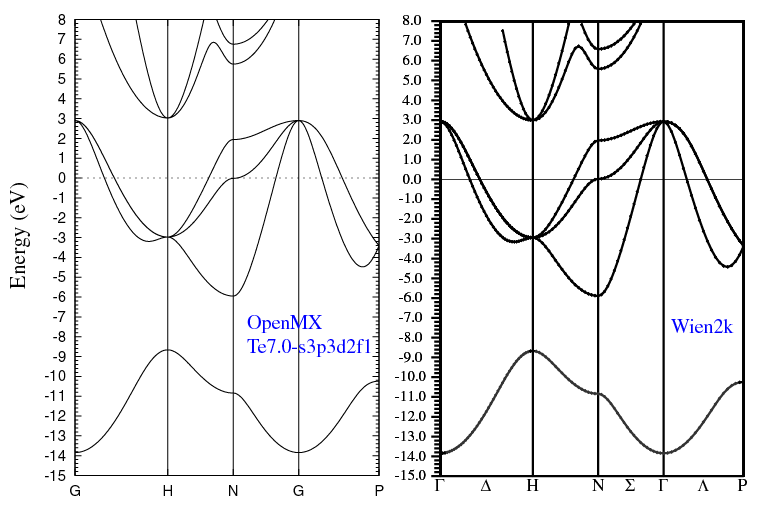### Supplementary information for the GGA (PBE13) pseudopotential